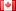# Pneumatic Air Gun Calculations

import matplotlib.pyplot as plt

Vm=var('Vm')

Patm=var('Patm')

Vc=var('Vc')

Ab=var('Ab')

Lb=var('Lb')

k=var('k')

cb=var('cb')

eng=var('eng')

eng2=var('eng2')

md=var('md')

Pc=var('Pc')

# Definitions

k = 1.4 Patm = 101235 cb = 0.261208

# m/s^2

Vm= 91.44 md=2 Pc=10 eng=0.618081

# Main Formula

solve(eng=(k-1)mdVm^2/(2PatmVc*(Pc-Pc^(1/k)),Vc)

Can anyone tell me why this is giving a syntax error and pointing to my variable definition Vm? It's really bugging me!2 No.2 RevisionDSM5242 ●21 ●68 ●112

# Pneumatic #Pneumatic Air Gun Calculations

 Calculations import matplotlib.pyplot as plt
plt #Variables Outlined Variables Outlined Muzzle Velocity Vm=var('Vm') Atmospheric Pressure Patm=var('Patm') Gas #Muzzle Velocity Vm=var('Vm') #Atmospheric Pressure Patm=var('Patm') #Gas Chamber Volume Vc=var('Vc') Barrel Area[Internal] Ab=var('Ab') Barrel Length Lb=var('Lb') Specific Volume Vc=var('Vc') #Barrel Area[Internal] Ab=var('Ab') #Barrel Length Lb=var('Lb') #Specific Heat of Air k=var('k') CB Air k=var('k') #CB Ratio[see table] cb=var('cb') formula variable eng=var('eng') 2nd table] cb=var('cb') #formula variable eng=var('eng') #2nd Formula Variable eng2=var('eng2') Mass Variable eng2=var('eng2') #Mass of the Projectile md=var('md') Operating Projectile md=var('md') #Operating Pressure in atmatm Pc=var('Pc') Pc=var('Pc') Definitions #Definitions k = 1.4 Patm = 101235 cb = 0.261208 m/s^2 0.261208 #m/s^2 Vm= 91.44 md=2 Pc=10 eng=0.618081eng=0.618081 Main Formula solve(eng=(k-1)mdVm^2/(2PatmVc*(Pc-Pc^(1/k)),Vc)#Main Formula solve(eng=(k-1)*md*Vm^2/(2*Patm*Vc*(Pc-Pc^(1/k)),Vc) 

Can anyone tell me why this is giving a syntax error and pointing to my variable definition Vm? It's really bugging me!3 retaggedFrédéricC4385 ●3 ●37 ●93

### What's Wrong With This?

#Pneumatic Air Gun Calculations
import matplotlib.pyplot as plt

#Variables Outlined

#Muzzle Velocity
Vm=var('Vm')
#Atmospheric Pressure
Patm=var('Patm')
#Gas Chamber Volume
Vc=var('Vc')
#Barrel Area[Internal]
Ab=var('Ab')
#Barrel Length
Lb=var('Lb')
#Specific Heat of Air
k=var('k')
#CB Ratio[see table]
cb=var('cb')
#formula variable
eng=var('eng')
#2nd Formula Variable
eng2=var('eng2')
#Mass of the Projectile
md=var('md')
#Operating Pressure in atm
Pc=var('Pc')

#Definitions
k = 1.4
Patm = 101235
cb = 0.261208
#m/s^2
Vm= 91.44
md=2
Pc=10
eng=0.618081

#Main Formula
solve(eng=(k-1)*md*Vm^2/(2*Patm*Vc*(Pc-Pc^(1/k)),Vc)


Can anyone tell me why this is giving a syntax error and pointing to my variable definition Vm? It's really bugging me!